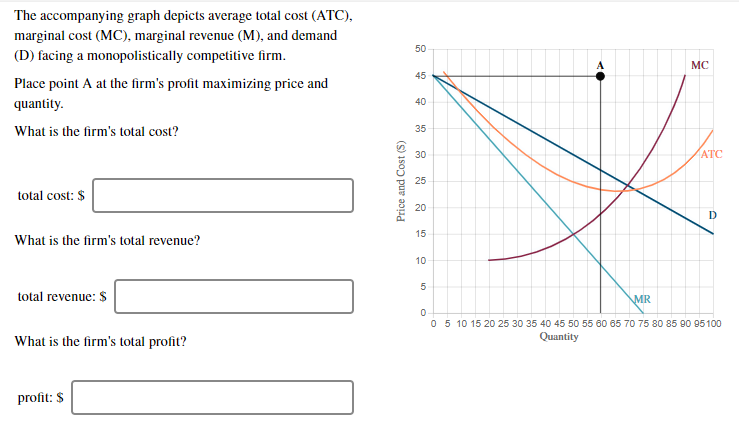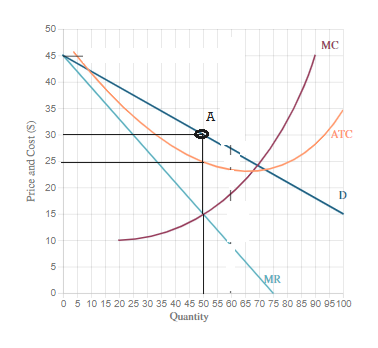# The accompanying graph depicts average total cost (ATC),marginal cost (MC), marginal revenue (M), and demand(D) facing a monopolistically competitive firm.50MC45Place point A at the firm's profit maximizing price and40quantity.35What is the firm's total cost?ATC3025total cost: \$2015What is the firm's total revenue?10total revenue: \$MR0 5 10 15 20 25 30 35 40 45 50 55 60 65 70 75 80 85 90 95100QuantityWhat is the firm's total profit?profit: \$Price and Cost (S)

Question
14 viewshelp_outlineImage TranscriptioncloseThe accompanying graph depicts average total cost (ATC), marginal cost (MC), marginal revenue (M), and demand (D) facing a monopolistically competitive firm. 50 MC 45 Place point A at the firm's profit maximizing price and 40 quantity. 35 What is the firm's total cost? ATC 30 25 total cost: \$ 20 15 What is the firm's total revenue? 10 total revenue: \$ MR 0 5 10 15 20 25 30 35 40 45 50 55 60 65 70 75 80 85 90 95100 Quantity What is the firm's total profit? profit: \$ Price and Cost (S) fullscreen
check_circle

Step 1

A monopolistic competitive firm maximises its profits, he will produce at a price where Marginal revenue (MR) = Marginal Cost (MC) and the perpendicular line that touches the demand curve above that is at price \$30 at which the output sold is 50 units. Point A is placed can be seen in the graph below:help_outlineImage Transcriptionclose50 MC 45 40 35 ATC 25 20 D 15 10 MR 0 5 10 15 20 25 30 35 40 45 50 55 60 65 70 75 80 85 90 95100 Quantity 30 LO Price and Cost (S) fullscreen
Step 2

The total cost can be obtained by multiplying ATC with the quantity produced at this price that is 50 units as we know, ATC = TC/Q

TC=ATC * Q = 25*50 = \$1250

Step 3

Total Revenue is equal to the price multiplied with quanti...

### Want to see the full answer?

See Solution

#### Want to see this answer and more?

Solutions are written by subject experts who are available 24/7. Questions are typically answered within 1 hour.*

See Solution
*Response times may vary by subject and question.
Tagged in